﻿ 龙格库塔间断有限元方法求解二维欧拉方程的多GPU加速实现

龙格库塔间断有限元方法求解二维欧拉方程的多GPU加速实现Accelerating the Runge-Kutta Discontinuous Galerking Method for Solving Two-Dimensional Flow on Multi GPUs

Abstract: It is time-consuming to use Runge-Kutta discontinuous Galerkin method to solver flow field. In this article, we use multi GPUs to accelerate computing of two-dimension NACA0012 airfoil. The flow mesh is divided into several blocks according to the number of GPUs. We specialize kernels with a one-element-per-thread strategy, and use MPI to communicate data among computing nodes. In the process of communication, we use CUDA stream and MPI non-blocking operation to overlap computation and communication. The result shows when compared with the serial CPU program, the speedup ratio of GPU code running on one, two, four GPUs is around 33, 59 and 108.

1. 引言

Klöckner A等人在单个GPU上构建了高效的高阶间断有限元解算器，并在三维四面体网格上求解了麦斯威尔方程，与同一代的CPU相比达到了近50倍的加速比  ；何晓峰等在单个GPU上，使用RKDG方法对二维欧拉方程进行了加速  ，与CPU相比获得了25倍的加速比；Dawei Mu等人在单个GPU上，使用间断有限元方法对三维弹性地震波方程进行了加速  ，并与CPU并行程序进行了比较，在单精度下相比1个、2个、4个、8个CPU并行程序获得的加速比分别为12.9、6.8和3.6。本文在此基于多GPU对RKDG方法进行加速。论文结构安排如下，我们首先介绍了龙格库塔间断有限元方法和CUDA程序概述，然后介绍了单GPU以及多GPU上基于CUDA的间断有限元方法求解，最后分别在一个、两个和四个GPU上进行了求解，统计了耗时，并与CPU结果进行了比较。

2. 龙格库塔间断有限元求解

$\left\{\begin{array}{l}{U}_{t}+F{\left(U\right)}_{x}+G{\left(U\right)}_{y}=0,\text{\hspace{0.17em}}\text{\hspace{0.17em}}\left(x,y\right)\in {R}^{2}\\ U\left(x,y,0\right)={U}_{0}\left(x,y\right).\end{array}$

$U=\left[\begin{array}{c}\rho \\ \rho u\\ \rho v\\ \rho E\end{array}\right]$ , $F\left(U\right)=\left[\begin{array}{c}\rho U\\ \rho {U}^{2}+P\\ \rho UV\\ \rho UH\end{array}\right]$ , $G\left(U\right)=\left[\begin{array}{c}\rho V\\ \rho UV\\ \rho {V}^{2}+P\\ \rho VH\end{array}\right]$

ρ，P，H和E分别为密度，压强，单位体积的总焓和单位体积的总能。

${U}_{h}={V}_{h}={V}_{h}^{N}\left\{p\in BV\cap {L}^{1}:{p|}_{K}\in {P}^{N}\left(K\right)\right\}$

K表示三角形剖分单元， ${P}^{N}\left(K\right)$ 表示单元K上次数不高于N的多项式。在三角形单元K上取基函数 $\left\{{v}_{0},{v}_{1},\cdots ,{v}_{m}\right\}$ ,则解函数 ${U}_{h}$ 可表示为：

${U}_{h}=\underset{p=0}{\overset{m}{\sum }}{U}_{K}^{\left(p\right)}\left(t\right)\cdot {v}_{p}\left(x,y\right)$ , $\left(x,y\right)\in K$

${v}_{0}\left(x,y\right)=1$

${v}_{1}\left(x,y\right)=\frac{x-{x}_{0}}{\sqrt{|{\Delta }_{0}|}}$

${v}_{2}\left(x,y\right)={a}_{21}\frac{x-{x}_{0}}{\sqrt{|{\Delta }_{0}|}}+\frac{y-{y}_{0}}{\sqrt{|{\Delta }_{0}|}}+{a}_{22}$${v}_{4}\left(x,y\right)={a}_{41}\frac{{\left(x-{x}_{0}\right)}^{2}}{|{\Delta }_{0}|}+\frac{\left(x-{x}_{0}\right)\left(y-{y}_{0}\right)}{|{\Delta }_{0}|}+{a}_{42}\frac{x-{x}_{0}}{\sqrt{|{\Delta }_{0}|}}+{a}_{43}\frac{y-{y}_{0}}{\sqrt{|{\Delta }_{0}|}}+{a}_{44}$

${v}_{5}\left(x,y\right)={a}_{51}\frac{{\left(x-{x}_{0}\right)}^{2}}{|{\Delta }_{0}|}+{a}_{52}\frac{\left(x-{x}_{0}\right)\left(y-{y}_{0}\right)}{|{\Delta }_{0}|}+\frac{{\left(y-{y}_{0}\right)}^{2}}{|{\Delta }_{0}|}+{a}_{53}\frac{x-{x}_{0}}{\sqrt{|{\Delta }_{0}|}}+{a}_{54}\frac{y-{y}_{0}}{\sqrt{|{\Delta }_{0}|}}+{a}_{55}$

$\frac{\text{d}}{\text{d}t}{U}^{h}={M}^{-1}L\left({U}^{h},t\right)$

3. GPU加速

3.1. Cuda概述

CUDA是显卡制造商Nvidia公司2007年推出的并行计算平台和编程模型，开发人员可以使用c/c++语言来为CUDA架构编写程序。CUDA提供host-device的编程模式以及非常多的接口函数和科学计算库，通过执行大量的线程来达到加速效果。CUDA通过线程格来管理线程。线程格包含多个线程块，线程块包含多个线程。线程格和线程块可以是一维、二维或者三维结构。本文使用一维线程块结构，其线程类似于一个二维的像素数组，如图1所示。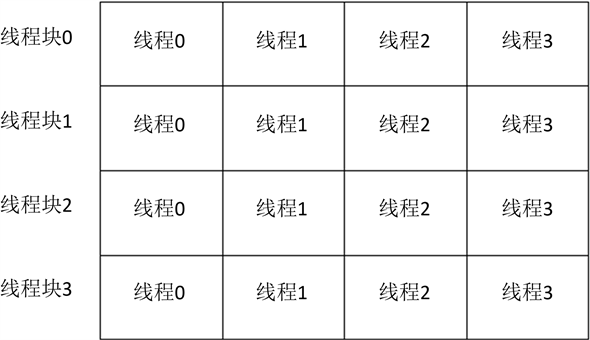Figure 1. Two-dimensional organazition of blocks and thread

3.2. 单GPU加速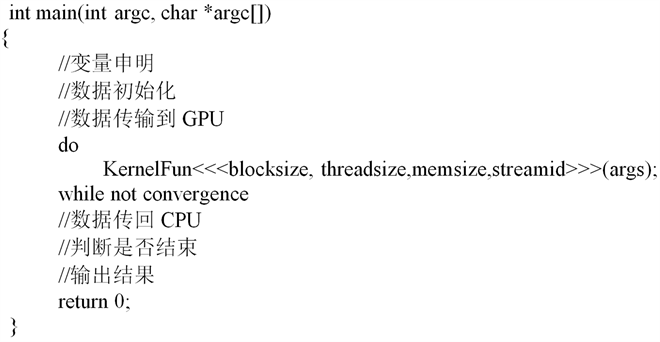3.3. 多GPU加速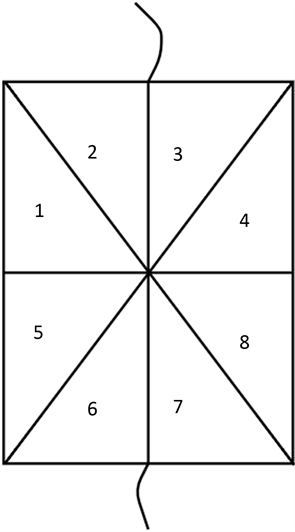Figure 2. Triangle unstructured mesh

4. 数值实验Figure 3. Triangle unstructured mesh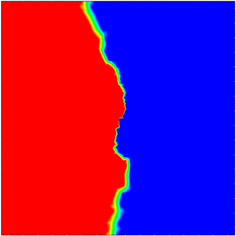Figure 4. Mesh divided into two parts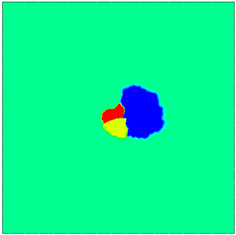Figure 5. Mesh divided into four partsTable 1. System resulting data of standard experimentFigure 6. Density contour map of solution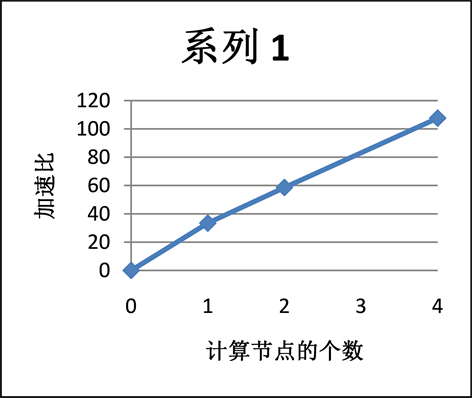Figure 7. Speedup ratio of different numbers of GPU relative CPU

5. 结论

 Qiu, J.X., Khoo, B.C. and Shu, C.-W. (2006) A Numerical Study for the Performance of the Runge-Kutta Discontinuous Galerkin Method Based on Different Numerical Fluxes. Journal of Computational Physics, 212, 540-565.
https://doi.org/10.1016/j.jcp.2005.07.011

 Sanders, J. and Kandrot, E. (2010) CUDA by Example: An Introduction to General-Purpose GPU Programming. Addison-Wesley Professional, .

 Klöckner, A., Warburton, T., Bridge, J., et al. (2009) Nodal Discontinuous Galerkin Methods on Graphics Processors. Journal of Computational Physics, 228, 7863-7882.
https://doi.org/10.1016/j.jcp.2009.06.041

 何晓峰, 程剑, 刘铁刚. 二维非结构网格上RKDG算法的CUDA解法器[C]//北京应用物理与计算数学研究所. 第十六届全国流体力学数值方法研讨会论文集: 2013年卷. 北京: CNKI, 2013.

 Mu, D.W., Chen, P. and Wang, L.Q. (2013) Accelerating the Discontinuous Galerkin Method for Seismic Wave Propagation Simulations Using the Graphic Processing Unit (GPU)—Single-GPU Implementation. Computers and Geosciences, 51, 282-292.
https://doi.org/10.1016/j.cageo.2012.07.017

 Cockburn, B. and Shu, C.W. (1991) The Runge-Kutta Local Projection $P^1$-Discontinuous-Galerkin Finite Element Method for Scalar Conservation Laws. ESAIM: Mathematical Modelling and Numerical Analysis, 25, 337-361.
https://doi.org/10.1051/m2an/1991250303371

 NVIDIA: NVIDIA CUDA C Programming Guide, NVIDIA Corporation, May, 2011.

 都志辉, 李三立, 审阅, 等. 高性能计算之并行编程技术——MPI并行程序设计[M]. 北京: 清华大学出版社, 2001.

Top